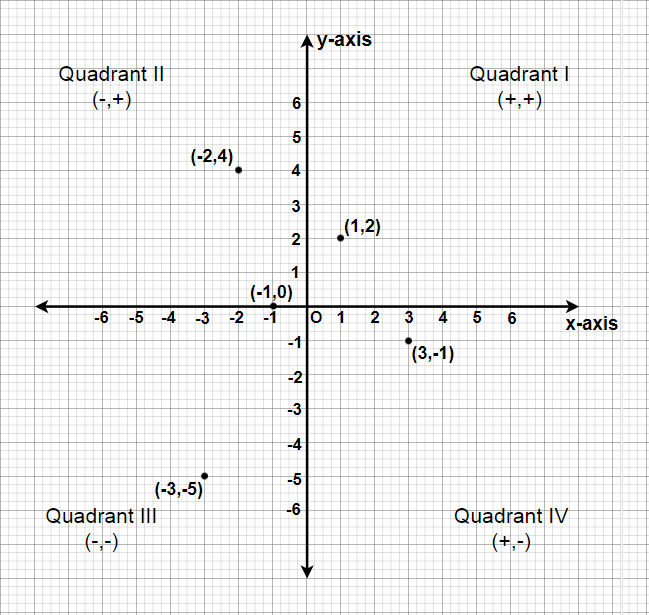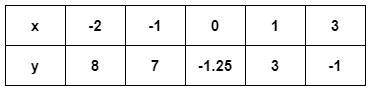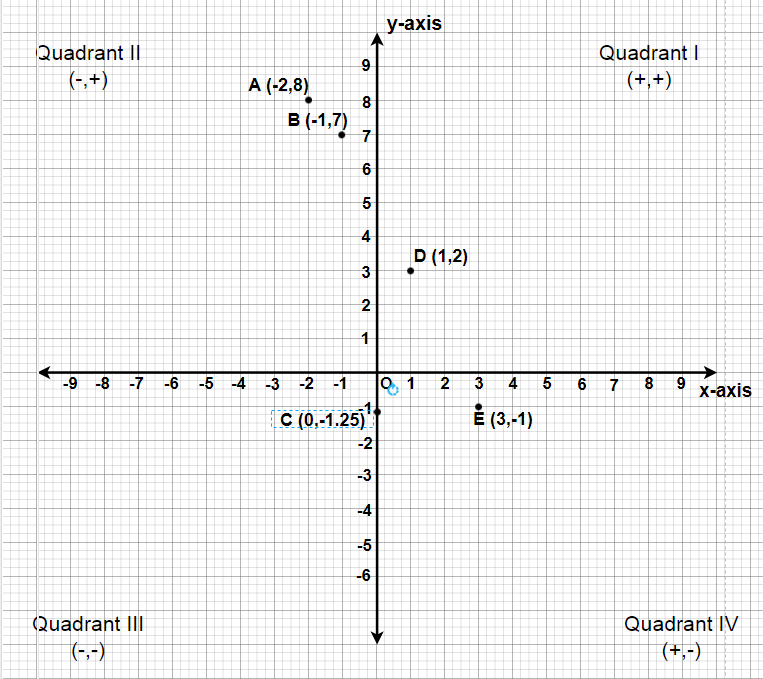# Class 9 NCERT Solutions – Chapter 3 Coordinate Geometry – Exercise 3.3

• Last Updated : 23 Feb, 2021

### Question 1: In which quadrant or on which axis do each of the points (– 2,  4), (3,  – 1), (– 1,  0), (1,  2) and (– 3,  – 5) lie? Verify your answer by locating them on the Cartesian plane.

Solution:Attention reader! All those who say programming isn't for kids, just haven't met the right mentors yet. Join the  Demo Class for First Step to Coding Coursespecifically designed for students of class 8 to 12.

The students will get to learn more about the world of programming in these free classes which will definitely help them in making a wise career choice in the future.

(i) The point (-2, 4) lies in IInd Quadrant in the Cartesian plane as x coordinate is negative and the y coordinate is positive.

(ii) The point (3, -1) lies in IVth Quadrant in the Cartesian plane as x coordinate is positive and the y coordinate is negative.

(iii) The point (-1, 0) lies on the negative x-axis and the value of x coordinate is negative.

(iv) The point (1, 2) lies in Ist Quadrant in the Cartesian as both x and y are positive.

(v) The point (-3,-5) lies in the IIIrd Quadrant in the Cartesian plane as both x and y are negative.

### Question 2: Plot the points (x, y) given in the following table on the plane, choosing suitable units of distance on the axes.Solution:

We have to plot these points A(-2, 8), B(-1, 7), C(0, -1.25), D(1, 3) and E(3, -1).
Steps we have to use to plot these points,

• Let 1 unit represents 1 cm.
• To plot (-2, 8), we take (-2) units on x-axis and (+8) units on y-axis. Now we can plot A (-2, 8), it will lie in quadrant-II.
• To plot (-1, 7), we take (-1) units on x-axis and (+7) units on y-axis. Now we can plot B(-1, 7), it will lie in quadrant-II.
• To plot (0, -1.25), we will proceed (-1.25) units under the x-axis on the y-axis and mark the plot as C(0, -1.25), it will lie on the negative side of y-axis.
• To plot (1, 3), we take (+1) unit on x-axis and (+3) units on y-axis. Now we can plot D(1, 3), it will lie in quadrant-I.
• To plot (3, -1), we take (+3) units on x-axis and (-1) unit on y-axis. Now we can plot the point E(3, -1), it will lie in quadrant-IV.My Personal Notes arrow_drop_up